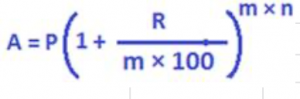Notation of terms in the above compound interest formula:

• P is the principal or the amount of money invested/deposited
• R is the rate of interest per annum compounded m times annually
• n is the no of years that the money is invested for
• A is the amount accruing after m × n units of times periods

Let us understand in the compound interest formula the term m clearly. The principle of continuous compounding or just compounding is a process of regular addition of interest to the principal at agreed intervals of time. During the time period of investment, interest is regularly added to the principal at specific intervals of time. This regular addition of interest to the principal, i.e. compounding can take place at different frequencies of time. In the above compound interest formula, the popular values of m, i.e. the intervals of time at the end of which interest will be added to the principal, are yearly, half-yearly, quarterly and monthly.

Below, the values of m for the respective compounding time periods are given:

• m = 1, if compounded annually or yearly, i.e. for every 12 months
• m = 2, if compounded half-yearly, i.e. for every 6 months,
• m = 4, if compounded quarterly, i.e. for every 3 months,
• m =12, if compounded quarterly, i.e. for every 12 months.

After substituting 1 in m for yearly compounding in the compound interest formula,we have the following well known compound interest formula for yearly compounding:

A very common mistake: It is very often assumed that simple interest for one year and compound interest for one year are both same, of course on a same principal and at the same rate of interest per annum. But, this is true only for annual compounding. Because, from the above compound interest formula for various periods of compounding, it is very clear that the two types of calculating interests, i.e. SI and CI, will generate a same amount of interest only when the frequency of compounding is yearly or annual

Consider the following example:

What interest amount will Rs. 1000 invested for 1 year at the rate of 5% per annum generate? Under Simple Interest, the interest amount is (1000 × 1 × 5)/100 = 50

Under Compound interest, from the compound interest formula for 1 year, the interest amount is 1000 (1 + (5/100))1 = 1000 × (105/100) = 1050

From the formula for amount, A = P + CI, we can see CI = A – P, i.e. CI = 1050 – 1000 = 50

Therefore, from this example, simple interest for one year and compound interest for one year are equal –for a same principal and interest rate, only when compounding takes place annually. Compound interest formula when rates of interest change every year. Sometimes the rate of interest can vary year after year. Suppose the rates of interest per annum are R1, R2, R3, and so on for the subsequent years. Then to find the amount generated under annual compounding, the compound interest formula is

Example:

What amount will accrue when Rs. 1000 is invested for 2 years at the rate of 5% p.a. for the first year and 6% for the second year, if compounding is done yearly?

Solution:

A = 1000 × (1 + (5/100)) × (1+(6/100)) = 1113